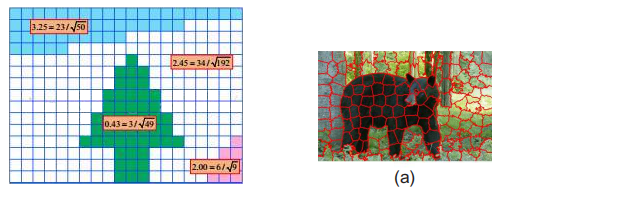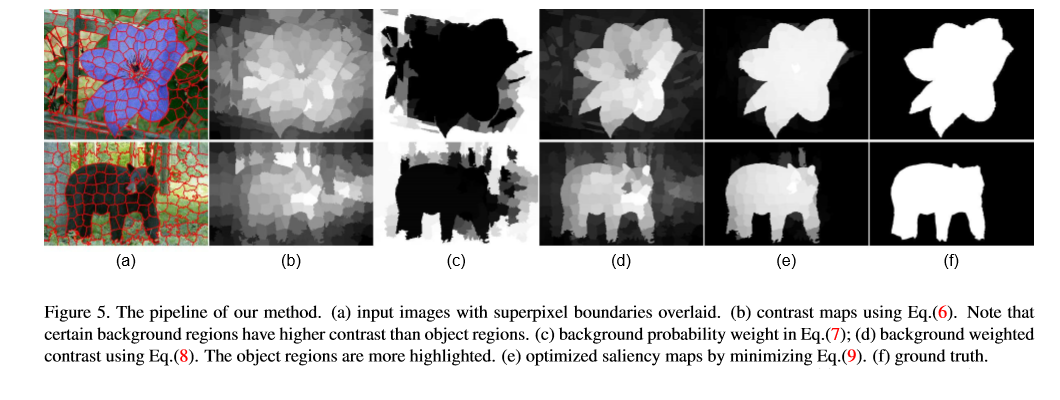# OpenCV—python 图像显著性检测算法—鲁棒背景检测

• 时间:

### 文章目录

• 一、鲁棒背景检测的显著性优化
• 二、代码演示

### 一、鲁棒背景检测的显著性优化

Saliency Optimization from Robust Background Detection

1.首先，我们提出一种鲁棒性的方法叫做背景相关性。它根据图像的边界来表征图像区域的空间布局，具有很强的鲁棒性。它有一个直观的几何解释，并提出独特的优势，这是以前的显着性方法所缺乏的。
2.我们提出一种优化框架原理来整合多个低层级线索，包括我们的背景方法，来得到干净并且规范的显著性图。
1. 显著性检测两种方法：对比度优先法边界优先法
2. 边界优先法的两个缺点：
（1）边界优先法简单的把所有边界都看作为背景。
（2）边界优先法的利用是启发式的。
3. 本文的工作提出新的方法解决上述两个问题：
（1）我们的第一个贡献是一种新的可靠的背景测量方法叫做边界相关性：一个图像块是背景仅当图像块着重属于图像边界。
（2）我们的第二个贡献是原则性框架将显著性估计看作是一种全局优化问题
代价函数前景线索 + 背景测量方法 + 平滑抑制

$BndCon(R) = \frac{ |\{ p|p \in R,p \in Bnd \} |}{\sqrt{|\{ p|p \in R\}|}} \tag{1}$
Bnd是图像边界块的集合，直观几何解释是边界上一个区域的周长整个区域的周长之比，或者说是面积的平方根之比。通常情况下背景的边界相关性很大，对象物体的边界相关性很小。1. 先通过图像分割进行区域分割， SLIC算法抽象处一组超像素（上图右）。然后通过提出连通度计算扩展到区域的计算。

2. 连接超像素，权值dapp为CIE-LAB之间的欧氏距离，dgeo是任意两边的测地线为最短路径。为了方便，dego=0。如下：
$d_{geo}(p,q) = \min_{p_1=p,p_2=p,...,p_n=p}\sum_{i=1}^{n-1}d_{app}(p_i,p_{i+1}) \tag{2}$

像素 p 的生成区域为 (N是超像素的数量)：
$\mathrm{Area}(p) = \sum_{i=1}^{N}\exp\left ( -\frac{d_{geo}^{2}(p,p_i)}{2\sigma_{clr}^2 } \right ) = \sum_{i=1}^{N}S(p,p_i) \tag{3}$

3. 边界长度：
$Len_{bnd}(p) = \sum_{i=1}^{N}S(p,p_i)\cdot \delta (p_i \in Bnd) \tag{4}$

4. 边界连通性：
$BndCon(p) = \frac{Len_{bnd}(p) }{\sqrt{\mathrm{Area}(p)}} \tag{5}$

• 优化点：超像素的权重
由于上述公式计算的结果可能对比度不高，于是作者又提出了一个背景对比度权重。
首先写出了超像素的权重：提高对比度的精度。
$Ctr(p) = \sum_{i=1}^{N}d_{\mathrm{app}}(p,p_i)\omega_{spa}(p,p_i)$

$\omega_{spa}(p,p_i) = \exp\left ( -\frac{d_{spa}^2(p,p_i)}{2\sigma_{spa}^2 } \right )$
同时还提出了背景概率：
$\omega_i^{bg} = 1-\exp\left ( -\frac{BndCon^2(p_i)}{2\sigma_{bndCon}^2 } \right )$
最终背景对比度权重为：
$\omega Ctr(p) = \sum_{i=1}^{N}d_{\mathrm{app}}(p,p_i)\omega_{spa}(p,p_i) \omega_i^{bg} \tag{8}$

• 显著性优化
用代价函数进行限制优化
$\underbrace{\sum_{i=1}^{N}w_i^{bg}s_i^2}_{background} + \underbrace{\sum_{i=1}^{N}w_i^{fg}(s_i-1)^2}_{background} + \underbrace{\sum_{i,j}w_{ij}(s_i-s_j)^2}_{smoothness} \tag{9}$

$w_{i,j} =\exp\left ( -\frac{d_{app}^{2}(p_i,p_j)}{2\sigma_{clr}^2 } \right ) + \mu \tag{10}$
最后通过最小二乘法进行优化得到最终的显著性区域。
描述了区域背景性和显著性目标性。前后几个效果图如下：### 二、代码演示

待续